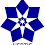## Tuesday, February 3, 2009

### Elearn Geometry Problem 241: Triangle with Equilateral triangles, CongruenceSee complete Problem 241 at:
gogeometry.com/problem/p241_triangle_equilateral_congruence_sas.htm

Level: High School, SAT Prep, College geometry

1.In triangles ABA' and C'BC we have C'B = AB & BC = BA'(sides of equilateral triangles) while the included angle in each case is angle (ABC+60) Thus the two triangles are congruent. So AA' = CC'
Ajit: ajitathle@gmail.com

2.See the drawing

- ∠CBC’=∠ABC+∠ABC’= ∠ABC+60°
- ∠ABA’= ∠ABC+∠CBA’= ∠ABC+60°
- =>∠CBC’=∠ABA’
- ΔABA’ is congruent to ΔCBC’ (SAS)
- Therefore AA’=CC’